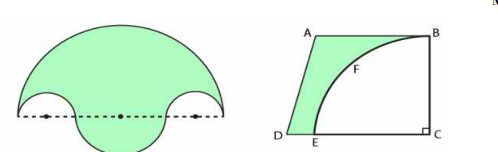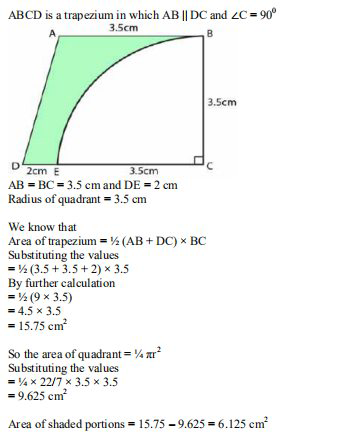# ML Aggarwal Solutions Class 9 Mathematics Solutions for Mensuration Exercise 16.3 in Chapter 16 - Mensuration

(a) In the figure (i) given below, the boundary of the shaded region in the given diagram consists of four

semicircular arcs, the smallest two being equal. If the diameter of the largest is 14 cm and of the smallest is

3.5 cm, calculate

(i) the length of the boundary.

(ii) the area of the shaded region.(b) In the figure (ii) given below, a piece of cardboard, in the shape of a trapezium ABCD, AB || CD and

∠BOD = 90^{\circ} , quarter circle BFEC is removed. Given AB = BC = 3.5 cm and DE = 2 cm. Calculate the area

of the remaining piece of the cardboard.

Perimeter for irregular shape is equal to the length of the boundary.

The area of irregular shapes refers to the area that the shape covers. Irregular forms are those that don't have the same number of sides or angles on all sides. To calculate the area of an irregular form, it must first be decomposed or divided into several common shapes, and then the areas of those shapes must be summed to obtain the total area.

(a) (i) We know that

Length of boundary = Circumference of bigger semi-circle + Circumference of small semi-circle + 2 ×

circumference of the smaller semi-circles(b) HereRelated Questions

Lido

Courses

Teachers

Book a Demo with us

Syllabus

Maths
CBSE
Maths
ICSE
Science
CBSE

Science
ICSE
English
CBSE
English
ICSE
Coding

Terms & Policies

Selina Question Bank

Maths
Physics
Biology

Allied Question Bank

Chemistry
Connect with us on social media!